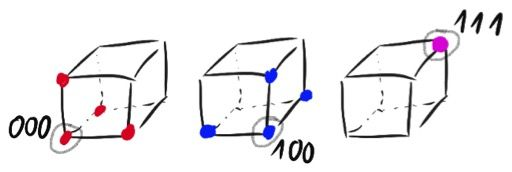시간 제한 메모리 제한 제출 정답 맞은 사람 정답 비율
5 초 1024 MB 0 0 0 0.000%

## 문제

Central European Regional Contest (CERC) is a contest famous for its interesting and always well-prepared tasks. One of these tasks was about finding a volume of a sum of three balls. Maybe it was a challenge 10 years ago, but nowadays contestants should not be bothered with so easy and standard problems. Instead of using 3D space, we will use $n$-dimensional hypercube. Obviously, it requires some definitions.

$n$-dimensional hypercube has $2^n$ vertices, each of them is represented by a sequence of $n$ coordinates which are either $0$ or $1$. For example, $3$-dimensional hypercube has $8$ vertices: 000, 001, 010, 011, 100, 101, 110, 111.

Ball with radius $r$ and center $s$ is a subset of vertices of hypercube which have distance at most $r$ to the vertex $s$. We compute the distance in Manhattan metric which means that vertex $p$ belongs to this ball if and only if coordinates of vertices $p$ and $s$ differ on at most $r$ positions.

Find the number of vertices which belong to the sum of three balls, i.e. number of vertices which belong to at least one of these balls. Print the result modulo $10^9+7$.

## 입력

First line of input contains one integer $n$ ($1 \leq n \leq 10\,000$), denoting number of dimensions.

Description of three balls follow. Each description takes one line and $i$-th line contains integer $r_i$ ($0 \leq r_i \leq n$) and binary word $s_i$ consisting of $n$ characters which are either $0$ or $1$. These are the radius and the center of the ball, respectively.

## 출력

You need to print one integer --- number of vertices belonging to sum of these three balls, modulo $10^9+7$.

## 예제 입력 1

3
1 000
1 100
0 111


## 예제 출력 1

7


## 예제 입력 2

5
2 10110
0 11010
1 00000


## 예제 출력 2

19


## 힌트

Explanation to first sample test: $3$-dimensional hypercube is just a mere cube. Following pictures show which vertices belong to following balls. Grey circle denotes center of a ball.First ball contains vertices 000, 100, 010, 001. Second ball contains vertices 100, 000, 110, 101. Third ball is just a single vertex 111. Sum of these balls contains $7$ vertices --- all of them except 011.